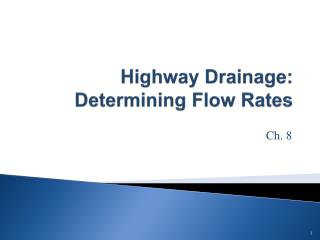DownloadDownload PresentationHighway Drainage: Determining Flow Rates

# Highway Drainage: Determining Flow Rates

Télécharger la présentation## Highway Drainage: Determining Flow Rates

- - - - - - - - - - - - - - - - - - - - - - - - - - - E N D - - - - - - - - - - - - - - - - - - - - - - - - - - -
##### Presentation Transcript

1. Objectives • Know how to determine peak flow using the rational method

2. Design Flow Methods • Rational method • TR-55 • Regression Equations • Historical Data

3. Methods-Smaller drainage areas • Rational Method • Drainage areas<200 acres • TR-55 (Technical Release-55) • Drainage areas < 2,000 acres

4. Methods-Larger drainage areas • TR-20 • Large drainage areas broken into subareas • HEC-RAS (Hydraulic Engineering Circular-River Analysis System)

5. Rational Method (metric)Q=kCIA • Q – peak discharge (m3/sec) • K- conversion factor= .00278 (cms-hr/ha-mm) • C-runoff coefficient (unitless) • I-rainfall intensity (mm/hr) • A-drainage area (hectares--100 square meters)

6. Rational Method (english)Q=kCIA • Q – peak discharge (cfs) • K (conversion factor)=1 (cfs-hr / in-acre) • C-runoff coefficient (unitless) • I-rainfall intensity (in/hr) • A-drainage area (acres)

7. Rational Method • Drainage area (previous lecture) • Time of concentration (need for I) • Rainfall intensity

8. Basic Steps to Estimate Peak Flow Rational Method • Determine Drainage Area (previous lecture) • Estimate time of concentration • Overland (sheet) flow • Shallow concentrated flow • Open channel flow • Choose a value of C • Select a frequency (return period) • Determine the average storm intensity from IDF Curve • Use rational method equation to estimate peak flow

9. Time of Concentration • Time required for water to flow from the most distant part of a drainage area to the drainage structure

10. Water can move through a watershed as: • Sheet flow (max of 300’; usually 100’) • Shallow concentrated flow • Open channel flow • Gutter • Ditch • Swale • Creek • Some combination of above

11. Example of Runoff Coefficient Table

12. Rational Coefficient C Must be weighted if you have different area types within the drainage area Drainage area = 8 acres: 2 acres; C=0.35 (residential suburban) 6 acres; C=0.2 (undeveloped-unimproved) Weighted C=[(2)(.35)+(6)(.2)]/8 = 0.24

13. Example: Determine the peak flow for a 100-acre watershed in central NY (mostly forested). Assume the intensity is 3” per hour. What is the peak flow if the watershed is developed into light industrial usage? Assume the intensity is 3” per hour.

14. Example-undeveloped: C=0.13 (reference table) I=3” per hour (given) A=100 acres Q=CIA = 39 cfs

15. Example-developed: C=0.65 (0.5+0.8)/2 I=3” per hour (given) A=100 acres Q=CIA = 195 cfs In reality, the peak could be higher, since the time of concentration would likely decrease (which would increase the intensity)

16. Detention Structures • Stores runoff temporarily and then releases it in a controlled manner to limit the peak flow leaving a site • Mitigates destructive effects of increased runoff • May improve water quality • May increase recharge http://www.tleng.net/Dentention_Pond.html#anchor_70

17. Detention Structures • Basically a tank or pond • Complex because water coming in (inflow hydrograph) is not constant • Water going out is not constant (single or multistaged outlet structure where Qout varies based on water elevation in the structure) • Water elevation in the structure is not constant